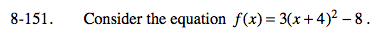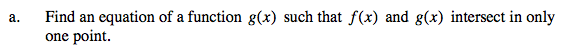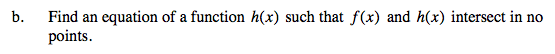Home > A2C > Chapter 8 > Lesson 8.2.3 > Problem8-151

8-151.
1. Consider the equation f(x) = 3(x + 4)2 − 8. Homework Help ✎

1. Find an equation of a function g(x) such that f(x) and g(x) intersect in only one point.

2. Find an equation of a function h(x) such that f(x) and h(x) intersect in no points.There are many possibilities here. You could find a horizontal line that doesn't intersect the parabola or an inverted parabola with a locator point in the fourth quadrant or below y = −8 in the third quadrant.

Use the eTool below to help solve the problem.
Click the link at right for the full version of the eTool: A2C 8-151 HW eTool